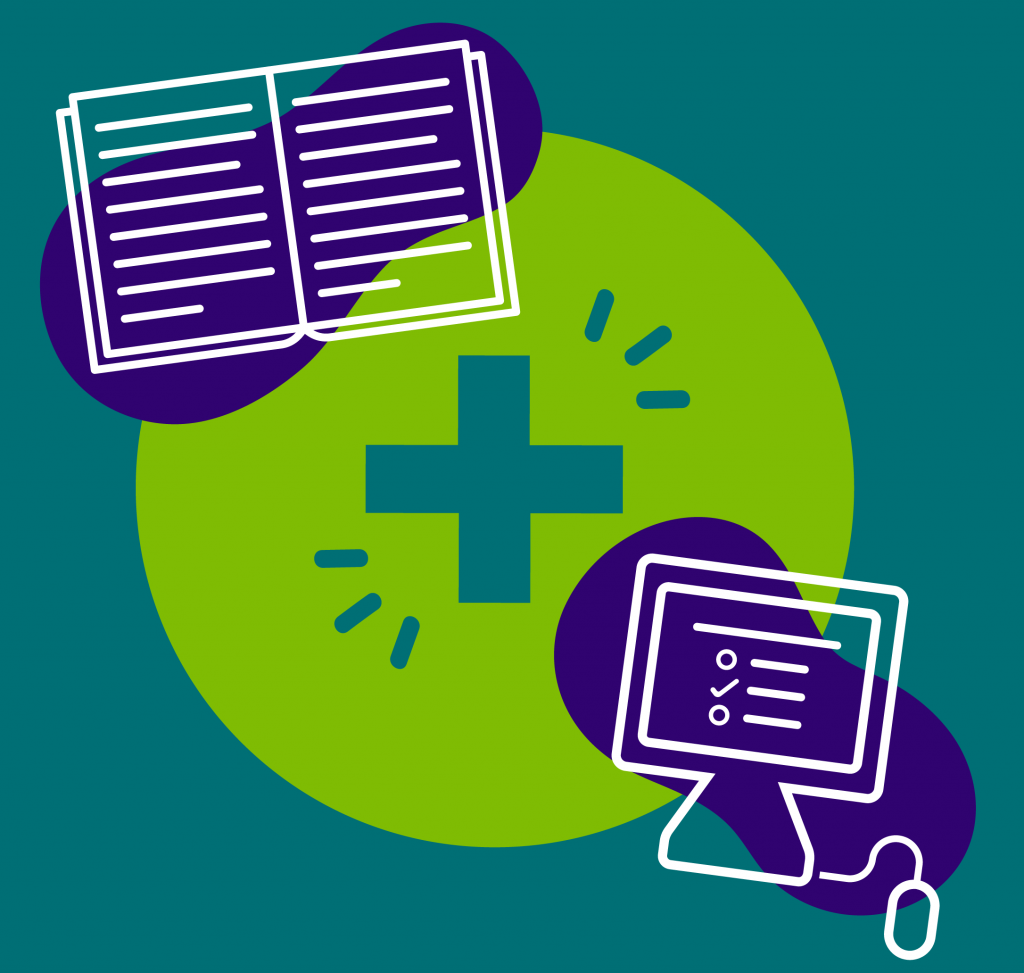###2nd grade students are now a part of our Blended Learning program, a combination of traditional pencil and paper, along with activities online.

A Grade Ahead’s 2nd grade Enrichment at Home worksheets extend the students’ understanding of numbers up to hundreds and builds their fluency in addition and subtraction. Students in our Enrichment at Home program apply these concepts consistently in word problems throughout the program. Curriculum skills include math skills, such as:

• Test-taking skills & strategies
• Word problems
• Logic
• Time, money, and calendar problems
• Standard and metric measurements
• Geometry

#### Don’t forget, your child can begin the curriculum at any time throughout the year. Start learning today!

You may also like 2nd Grade English.

Get a sample instantly!
• ## Curious about our material? Get a sample instantly!

• This field is for validation purposes and should be left unchanged.

June
Month 1
Curriculum Topics
Counting; Place value
Numerical Path
Carry 1:
Examples: 67 + 25 = ? and 38 - 28 = ?
July
Month 2
Curriculum Topics
Rounding; Estimation
Numerical Path
Carry 2:
Examples: 145 + 67 = ? and 198 - 45 = ?
August
Month 3
Curriculum Topics
Money
Numerical Path
Carry/Borrow 1:
Examples: 87 + 23 = ? And 64 - 29 = ?
September
Month 4
Curriculum Topics
Numerical Path
Carry/Borrow 2:
Examples: 385 + 67 = ? and 387 - 99 = ?
October
Month 5
Curriculum Topics
Geometry
Numerical Path
Mental Math:
Examples: 912 - 2 = ? and 872 + 5 = ?
November
Month 6
Curriculum Topics
Fractions; Calendar
Numerical Path
Rounding:
Examples: 269 + 86 -> 270 + 90
December
Month 7
Curriculum Topics
Time
Numerical Path
Carry/Borrow 3:
Examples: 537 + 133 = ? and 809 - 178 = ?
January
Month 8
Curriculum Topics
Tables; Bar and line graphs
Numerical Path
Carry/Borrow 4:
Examples: 944 - 890 = ? and 845 - 787 = ?
February
Test Prep Month
Curriculum Topics
Numerical Path
Carry/Borrow 5:
Examples: 112 + 138 (horizontal) = ?
March
Month 9
Curriculum Topics
Length; Weight
Numerical Path
Carry/Borrow 6:
Example: 193 + 362 + 28 = ? And 792 + 328 + 64 = ?
April
Month 10
Curriculum Topics
Liquid volume; Algebraic thinking; Multiplication
Numerical Path
Algebraic Thinking 1:
Examples: 207 + _ = 385 and _ - 187 = 135
May
Month 11
Curriculum Topics